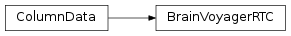# mvpa2.misc.bv.base.BrainVoyagerRTC¶class `mvpa2.misc.bv.base.``BrainVoyagerRTC`(source)

IO helper to read BrainVoyager RTC files.

This is a textfile format that is used to specify stimulation protocols for data analysis in BrainVoyager. It looks like

FileVersion: 2 Type: DesignMatrix NrOfPredictors: 4 NrOfDataPoints: 147

“fm_l_60dB” “fm_r_60dB” “fm_l_80dB” “fm_r_80dB” 0.000000 0.000000 0.000000 0.000000 0.000000 0.000000 0.000000 0.000000 0.000000 0.000000 0.000000 0.000000

Data is always read as `float` and header is actually ignored

Attributes

 `ncolumns` Returns the number of columns. `nrows` Returns the number of rows.

Methods

 `clear`(() -> None.  Remove all items from D.) `copy`(() -> a shallow copy of D) `fromkeys`(...) v defaults to None. `get`((k[,d]) -> D[k] if k in D, ...) `has_key`((k) -> True if D has a key k, else False) `items`(() -> list of D’s (key, value) pairs, ...) `iteritems`(() -> an iterator over the (key, ...) `iterkeys`(() -> an iterator over the keys of D) `itervalues`(...) `keys`(() -> list of D’s keys) `pop`((k[,d]) -> v, ...) If key is not found, d is returned if given, otherwise KeyError is raised `popitem`(() -> (k, v), ...) 2-tuple; but raise KeyError if D is empty. `select_samples`(selection) Return new ColumnData with selected samples `setdefault`((k[,d]) -> D.get(k,d), ...) `toarray`() Returns the data as an array `tofile`(filename[, header, header_order, sep]) Write column data to a text file. `update`(([E, ...) If E present and has a .keys() method, does: for k in E: D[k] = E[k] `values`(() -> list of D’s values) `viewitems`(...) `viewkeys`(...) `viewvalues`(...)

Read and write BrainVoyager RTC files.

Parameters: source : str Filename of an RTC file

Attributes

 `ncolumns` Returns the number of columns. `nrows` Returns the number of rows.

Methods

 `clear`(() -> None.  Remove all items from D.) `copy`(() -> a shallow copy of D) `fromkeys`(...) v defaults to None. `get`((k[,d]) -> D[k] if k in D, ...) `has_key`((k) -> True if D has a key k, else False) `items`(() -> list of D’s (key, value) pairs, ...) `iteritems`(() -> an iterator over the (key, ...) `iterkeys`(() -> an iterator over the keys of D) `itervalues`(...) `keys`(() -> list of D’s keys) `pop`((k[,d]) -> v, ...) If key is not found, d is returned if given, otherwise KeyError is raised `popitem`(() -> (k, v), ...) 2-tuple; but raise KeyError if D is empty. `select_samples`(selection) Return new ColumnData with selected samples `setdefault`((k[,d]) -> D.get(k,d), ...) `toarray`() Returns the data as an array `tofile`(filename[, header, header_order, sep]) Write column data to a text file. `update`(([E, ...) If E present and has a .keys() method, does: for k in E: D[k] = E[k] `values`(() -> list of D’s values) `viewitems`(...) `viewkeys`(...) `viewvalues`(...)
`toarray`()

Returns the data as an array